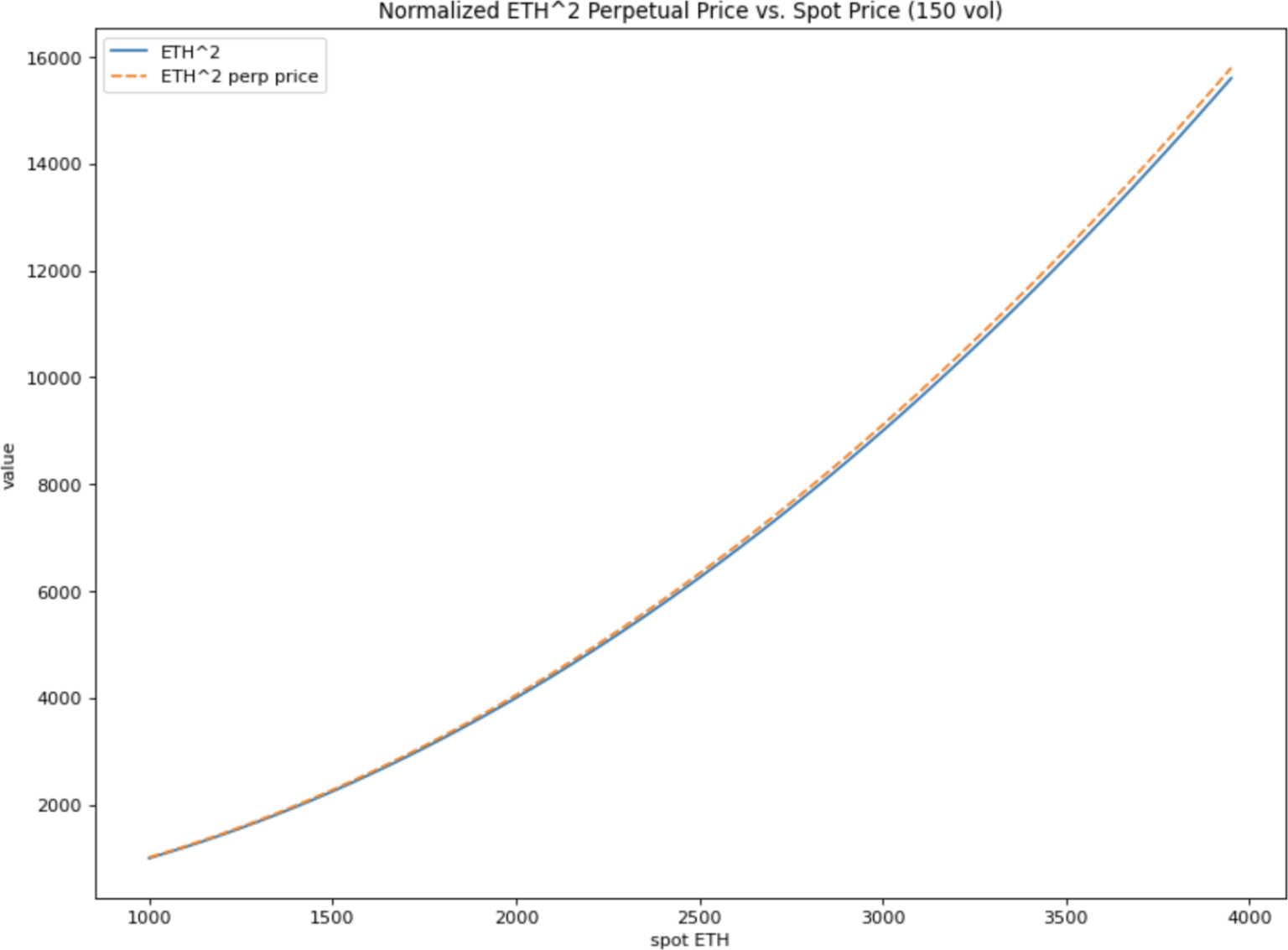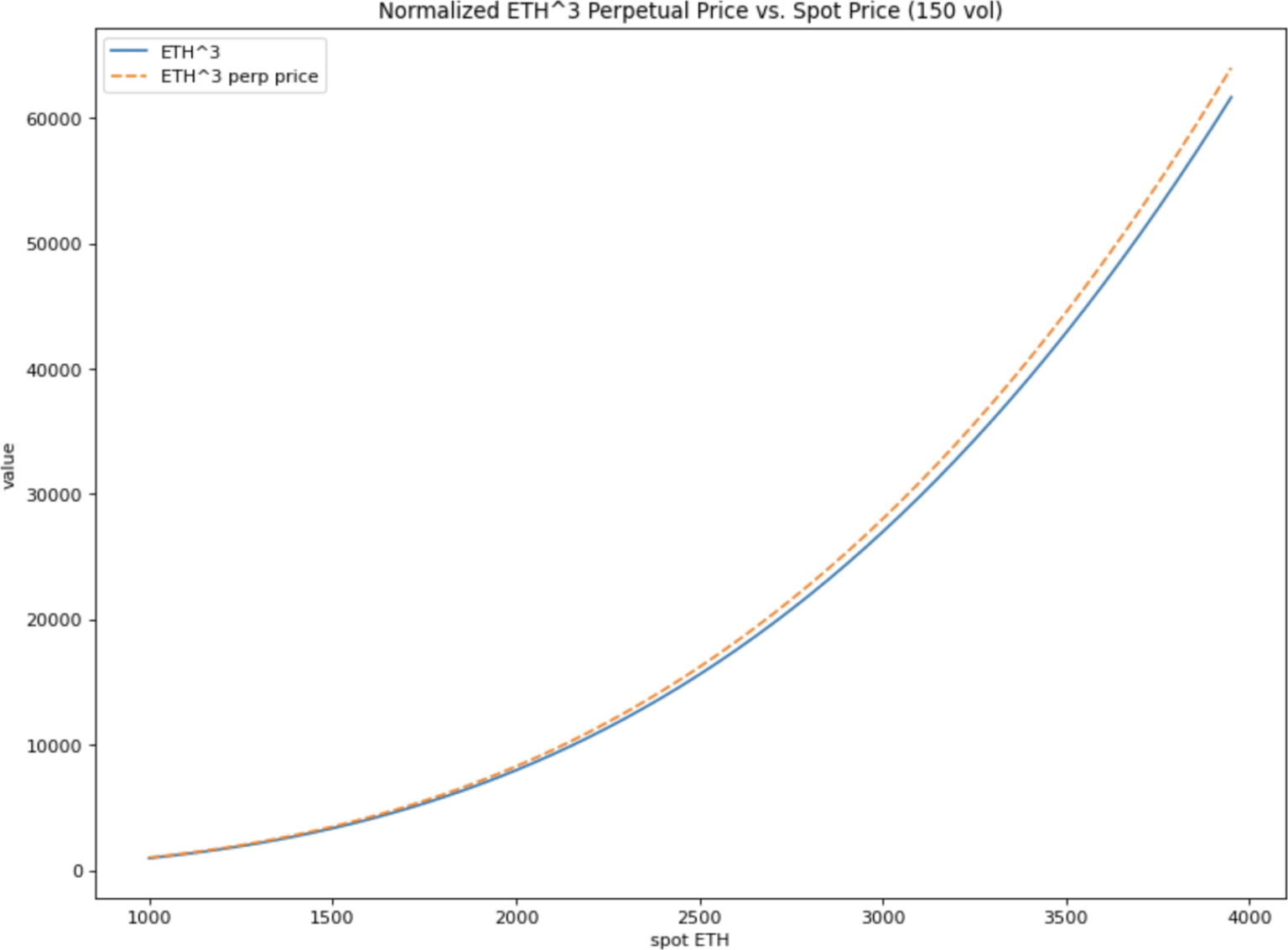## 定价

### 推导

$S^pe^{t\frac {p-1}{2}(2r+pv^2)}$

$S^p\frac {1}{2e^{-f\frac {p-1}{2}(2r+pv^2)}-1}$

$\text {MARK}-\text {INDEX} = S^P (\frac {1}{2e^{-f\frac {p-1}{2}(2r+pv^2)}-1}-1)$

### 收敛性

$\frac {e^{f\frac {p-1}{2}(2r+pv^2)}}{2} < 1$

## 例子

### ETH^2 乘方永续合约### ETH^3 乘方永续合约ETH^3 乘方永续合约的价格为 $$S^3\frac {1}{2e^{-f（2r+pv^2）}-1}$$。